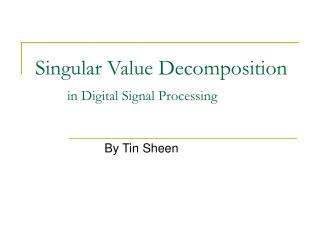DownloadDownload PresentationSingular Value Decomposition in Digital Signal Processing

# Singular Value Decomposition in Digital Signal Processing

Download Presentation## Singular Value Decomposition in Digital Signal Processing

- - - - - - - - - - - - - - - - - - - - - - - - - - - E N D - - - - - - - - - - - - - - - - - - - - - - - - - - -
##### Presentation Transcript

1. Signals • Flow of information • Measured quantity that varies with time (or position) • Electrical signal received from a transducer (microphone, thermometer, accelerometer, antenna, etc.) • Electrical signal that controls a process

2. SVD background • The Singular Value Decomposition (SVD) of a rectangular matrix A is a decomposition of the form • A = U S VT • U and V are orthogonal matrices • and S is a diagonal matrix • The singular vectors form orthonormal bases, and the important relation • A vi = si ui • shows that each right singular vectors is mapped onto the corresponding left singular vector, and the "magnification factor" is the corresponding singular value.

3. What is Digital Signal Processing? • Digital signal processing (DSP) is the study of signals in a digital representation and the processing methods of these signals. • DSP includes subfields like: audio signal processing, control engineering, digital image processing and speech processing.

4. Noise reduction • The SVD has also applications in digital signal processing, e.g., as a method for noise reduction. The central idea is to let a matrix A represent the noisy signal, compute the SVD, and then discard small singular values of A. It can be shown that the small singular values mainly represent the noise, and thus the rank-k matrix Akrepresents a filtered signal with less noise.

5. SVD’s usefulness • SVD is sufficient and is the most optimal in given image. • It is packed with energy in a given number of transformation coefficients is maximized. • Easy to calculate.

6. Normal vs Noised Image

7. Block SVD filtering algorithm • Let the original, non-corrupted image F be represented as KxL matrix. Adding the noise to the original F image will produces the noised image G of the same size • G = F + N • Where N = random KxL noise field • G will be divided into bxb matrix image • Gij = Uij Sij VijT where i = 1,2,3,4,…k; j =1,2,3,4,…,l • Where Uij is the left singular vector, Sij is the diagonal, and Vij is the right singular vector • From here we can calculate the mean value of the rank (rank obtained from Gij * Sij)

8. SVD’s calculation • The proposed algorithm has two basic steps: first, the noise variance (variance of a random variable (or somewhat more precisely, of a probability distribution) is a measure of its statistical dispersion, indicating how its possible values are spread around the expected value) is estimated, • And then filtering is performed on singular values and vectors. Noised image is divided into square blocks of size bxb. For each block the singular value decomposition is performed. In the consequent step, the average sum of the last t singular values is calculated over all image

9. Noised calculation • Previously calculated SVD of image blocks will now be used for filtering. • First step in filtering is decreasing of noised singular value si jrfor every image block : • ŝi jr = si jr – p1 * ns *w(r) • where ŝi jris filtered singular value,p1is image dependent parameter and w(r) is a weighting function that determines percentage of estimated noise variance to be subtracted from noised • singular value si jr. The weighting function used in this work is chosen to be:

10. Implementation of DSP

11. Conclusion • Digital signal processing is a stealth technology. It is the core enabling technology in everything from your cell phone to the Mars Rover. It goes much further than just enabling a one-time breakthrough product. It provides ever-increasing capability; compare the performance gains made by dial-up modems with the recent performance gains of DSL and cable modems. Remarkably, digital signal processing has become ubiquitous with little fanfare, and most of its users are not even aware of what it is.